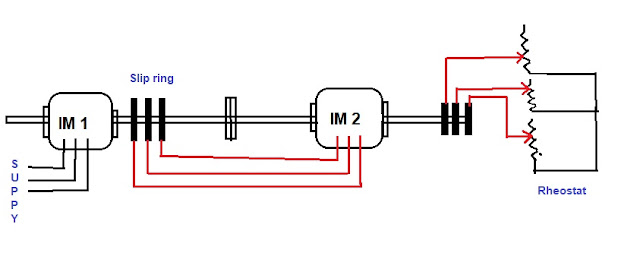# Speed Control of Induction Motor-Methods

A three-phase AC induction motor is a constant speed motor as a dc shunt motor. But the speed of DC shunt motor can be changed smoothly simply using rheostats. This maintains the speed regulation and efficiency of DC shunt motor. But in the case of 3 phase induction motors very difficult to control speed smoothly. if the speed controlled by using some methods, the performance of the induction motor in terms of its power factor,  efficiency, etc. gets badly affected. as we know induction motor widely being used, in domestic as well as in industrial application hence speed control of induction motor is necessary and achieved by using various methods like changing frequency, V/f control or frequency control, changing the number of poles, by emf injection method. Different speed control methods of induction motor are explained below.

## Speed control by changing frequency

This method of motor speed control is impractical for most of the applications because the frequency of the supply must remain fixed. In some cases where the rotor load is the only connected to the generator set, the speed of prime mover of a generator may be varied to achieve change in supply frequency and hence change the motor speed.

## Speed control by changing the number of poleschanging poles

Speed control of induction motor by changing the pole circuit diagram shown above From the synchronous speed equation, we can conclude that change in the number of poles by making a change in the stator winding connection with the help of suitable switching method we can achieve speed control.

Two separate windings are on the stator producing the same numbers of poles, one winding creates two N- poles, and another winding two S- poles. As shown in the figure, This arrangement gives four poles and speed at 50Hz is 1500 rpm.induction motor speed equation

(120 x 50)/ 4

=1500

If the connection of one of the winding makes reversed there will be 4 N poles and 4 S-poles depending upon which winding connection is reversed. But in between these 4 poles, other 4 poles of opposite polarity will be created.eight poles

Thus stator now has eight poles and its synchronous speed is 750RPM.

## Speed Control by changing slip

Change in slip is made by introducing an external resistance in the rotor circuit of the wound type rotor, but this produced an effect on the efficiency of the Induction motor and make speed regulation poor.

## speed control of induction motor by emf injection method

### supplying counter-EMF to the rotor at slip frequency

To supply counter EMF by this method required auxiliary commutating machine which injects EMF into the rotor at rotor frequency through slip ring arrangement

this method of speed control had the advantages of wide range adjustment in speed without affecting the efficiency of a motor

### Supplying EMF to the rotor at the supply frequency

This method requires a commutator for the Rotor

## Control speed by cascade connectiontandem connection

This method of speed control of Induction Motor also known as a tandem connection.When multiple speeds required motor is operated in cascade. In cascade connection, two Motors are rigidly coupled to the same shaft by using gears.

The stator of the first motor is connected to the main supply while stator of second cascade-connected Motor is fed from the rotor winding of the first motor. After that, the rotor circuit of the second motor is connected to the slip ring circuit so resistance may be introduced while starting and for additional speed control when running

Related terms